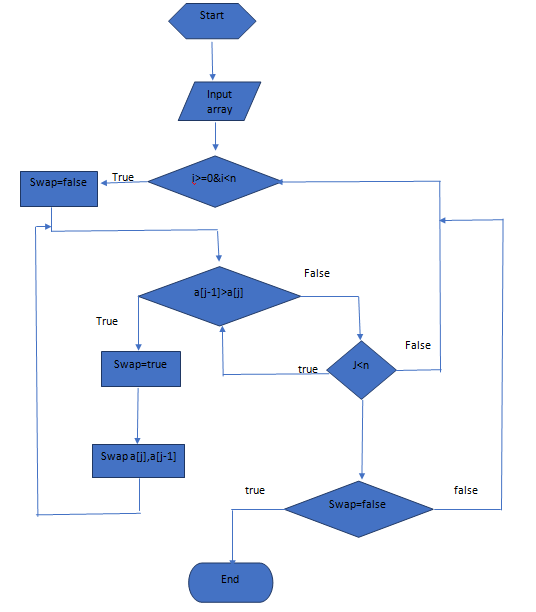# Bubble sort Algorithm, Flow Chart and C++ Code

In this article, we are going to learn about Bubble Sort, its algorithm, flow chart and c++ program to implement bubble sort.
Submitted by Raunak Goswami, on August 09, 2018

We are going to look at the algorithm of one of the simplest and the easiest sorting technique. since algorithm are language independent so you can use this algorithm to write your code in any language that you prefer.

The basic logic behind this algorithm is that the computer selects the first element and performs swapping by the adjacent element if required based on the kind of sorting i.e. ascending and descending till it reaches the last element this is known as a pass. The computer performs multiple such passes till all the elements are sorted or no more swapping is possible.

## Algorithm for bubble sort

```    Bubble Sort(a[],n)
For i=0 to n-1
Swap=false
For j=i+1 to n
if a[j-1] >a[j]
Swap(a[j-1],a[j])
Swap=true
Break if not swapped
```

To help you understand better you can look at the flowchart for the bubble sort given below:

## Flow chart for bubble sortNow, let us write a C++ code to sort 5 elements using bubble sort. The following code is written for ubuntu users. For windows users just replace #include <iostream> with #include<iostream.h> and skip using namespace std; and include conio header file.

### Code for bubble sort

```#include <iostream>
using namespace std;

int main()
{
int a;
int temp;
for(int i=0;i<5;i++)
{
cin>>a[i];
}

for(int j=0;j<4;j++)
{
for(int k=j+1;k<5;k++)
if(a[j]>a[k])
{
temp=a[k];
a[k]=a[j];
a[j]=temp;
}
}

cout<<"the elements after sorting"<<endl;
for(int i=0;i<5;i++)
cout<<a[i]<<endl;

return 0;
}
```

Output

```    4
5
3
2
1

the elements after sorting
1
2
3
4
5
```

What's New (MCQs)

Top Interview Coding Problems/Challenges!

IncludeHelp's Blogs

Languages: » C » C++ » C++ STL » Java » Data Structure » C#.Net » Android » Kotlin » SQL
Web Technologies: » PHP » Python » JavaScript » CSS » Ajax » Node.js » Web programming/HTML
Solved programs: » C » C++ » DS » Java » C#
Aptitude que. & ans.: » C » C++ » Java » DBMS
Interview que. & ans.: » C » Embedded C » Java » SEO » HR
CS Subjects: » CS Basics » O.S. » Networks » DBMS » Embedded Systems » Cloud Computing
» Machine learning » CS Organizations » Linux » DOS
More: » Articles » Puzzles » News/Updates